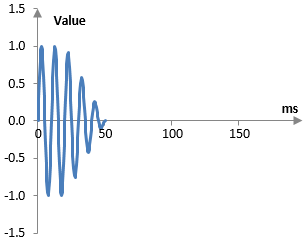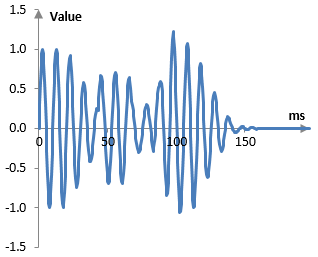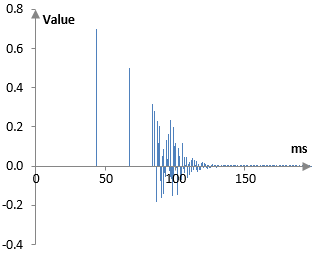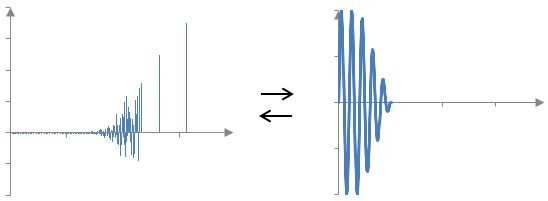# Impulse reverb

An impulse reverb, also known as "convolution reverb", is an effect that creates artificial reverberations by convolving the impulse response of actual reverberations with the recorded sound.

If we have a space (a room or a hall) with nice natural reverberations, we can record the dry (without reverberations) and reverberated versions of the same sound. These two signals can be used to compute the impulse response of the natural reverberations as in the example below. The impulse response can then be convolved with any recorded sound to produce an artificial reverb that models the natural reverberations. The fact that the impulse response is convolved with the signal to produce an artificial reverb gives rise to the name "convolution reverb".

## Creating impulse reverbs with sine sweeps and frequency domain deconvolution

One example of this process is presented in the topic Sine sweep. Sine sweeps are good candidates for the sound to be played and recorded, because computing the impulse of the reverb from the resulting signal is easy – the resulting signal is convolved with the sine sweep inverted along the time line, usually with some adjustments to its amplitude. Since the convolution can be computationally intensive, if done in the time domain, it can be performed in the frequency domain.

## A theoretical example computation of the impulse reverb with a transient sound

The example below is another example. Rather than using a sine sweep, it uses a short (transient) sound. This is not the preferred method but is easier to understand.

Short sounds were the early candidates for sounds to be played and recorded, because it was assumed that it would be easier to compute the impulse of the reverb if the original signal resembled an impulse.

Suppose that we have two recordings of the same drum hits – one dry, without reverberation and one with the natural reverberations of the room. The following graph is an example of what the recorded dry drum hit signal may look like.The reverberated drum hit may be as in the graph below.(Both graphs above are not actual recordings, but simply examples that will suffice for the purpose of the computations below. The drum hit above is simply a decaying sine wave. The reverberated drum hit uses a tapped delay line and a simple Shroeder reverb. The tapped delay line has two taps with delays of 43 ms and 67 ms and decays of 0.7 and 0.5. The Shroeder reverb has two Shroeder all pass filters with delays of 3.7 ms and 1.2 ms and decays of 0.7 and 0.65 and one feedforward comb filter with delay of 11 ms and decay of 0.7.)

If we have the dry and reverberated signals, x(k) and y(k) respectively, we can compute the impulse response of the reverb, a(k). Since both signals are finite, the first sample of the convolution y(0) is computed only from one sample of the impulse response a(0) and one sample of the drum hit x(0), the second sample of the convolution is computed from two samples of each of the impulse response and the drum hit, and so on. We have

$$a(0) = \frac{y(0)}{x(0)}$$ $$a(1)=\frac{y(1)-a(0)x(0)}{x(1)}$$ $$a(2)=\frac{y(2)-a(0)x(0)-a(1)x(1)}{x(2)}$$ $$...$$

The resulting impulse response with the example above is shown in the following graph.(In this particular example, the first two notches of the impulse response are the output of the tapped delay line. The remainder is the output of the Shroeder reverb, in which the all pass filter create a multitude of successful repetitions and the one peak in the middle of these multiple repetitions is created by the comb filter. The impulse response above omits the dry signal and presents only the reverberated portion).

## Computing the impulse response in practice

Computing the impulse response of the natural reverberations in practice is more difficult. It may not be possible to separate the dry from the reverberated signal precisely. Even if we can use two microphones to record the dry and reverberated sound, it is likely that: 1) some of the reverberations will appear into the microphone that should be recording only the dry sound; and 2) the two microphones may record the dry signal differently.

If the differences are minor, the computation above may still work. The following graph shows the impulse response from above (blue) and an impulse response (red) computed with the same reverberated recorded signal, but a different dry signal (a dry signal with slight random errors between -0.02 and 0.02 in each sample).In some cases, as in the one here, depending on the error, the computation above may produce an impulse response, the tail of which does not decay or even becomes infinitely large. In such cases, the impulse response may be decayed artificially.

Alternatively, the impulse response may be computed with an optimization. The optimization problem will look for the impulse response that, given the recorded dry drum sound, produces a reverberated sound that is as close to the recorded reverberated sound as possible. It is possible to set up optimization problems that enforces appropriate impulse response decay. One could, for example, set up an optimization, in which the reverb is already modeled structurally (e.g., with the tapped delay line and the Shroeder reverb) and only the parameters of that structural model are allowed to change, but not the structure itself.

## Applying the impulse response (convolving)

To reverberate any sound, the impulse response is convolved with that sound as in the graph below.If a(k) is the impulse response of length N + 1 and x(k) is the dry signal, the convolution computes the reverberated signal y(k) with the formula

$$y(k)=(a*x)(k)=\sum_{n=0}^{N} a(n)\,x(k-n)$$

### School 2019

Now all is clear, thanks for an explanation.

### You're my only hope guys =(

I love all your posts. You have done great job

### Who can help me with this? (((

Whoa! This is by far the most helpful thing on the subject I have ever found. Thanks for your hard work.

### Filtered HTML

• Freelinking helps you easily create HTML links. Links take the form of [[indicator:target|Title]]. By default (no indicator): Click to view a local node.